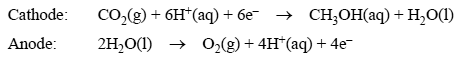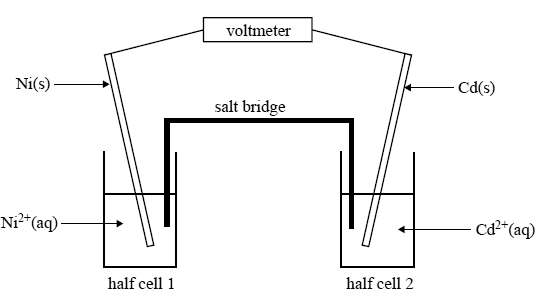Electrolysis 2008 VCE A research chemist is working on developing a catalytic electrode that makes possible the formation of methanol (CH3OH) in an electrolytic cell using carbon dioxide from the air. The electrode reactions in the electrolytic cell areThe aim of the research is to use electricity generated from a solar cell to produce the methanol. The resulting methanol could then be extracted and used as a fuel by burning it in air. Give a balanced equation for the complete combustion of methanol with oxygen. Solution Give the value in kJ mol–1, and sign, of ΔH for the reaction you have written above. Solution A particular experimental electrolytic cell operates for 24.0 hours at a constant current of 25.5 A. Calculate the amount of electricity, in coulomb, that passes through the cell. Solution Calculate the mass, in grams, of methanol that forms during that time, assuming that all the electricity that passes through the cell is used to produce methanol. Solution In practice, it is found that less than the calculated amount of methanol is actually produced in this experiment. Given that the experimental readings of current, time and mass of methanol obtained are accurate, give one reason why the amount of methanol is lower than predicted. Solution . `